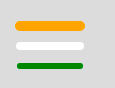GeeksforGeeks App
Open AppBrowser
Continue

The strokeWeight() function in p5.js is used to set the width of the stroke used for lines, points and the border around shapes. The stroke weight is set by using pixels.

Syntax:

`strokeWeight(weight)`

Parameters: This function accepts a single parameter weight which stores the weight (in pixels) of the stroke. The below programs illustrate the strokeWeight() function in p5.js:

Example 1: This example uses strokeWeight() function to set the width of the stroke.

## javascript

 `function` `setup() {` `    ``// Create Canvas of given size``    ``createCanvas(380, 170);``}` `function` `draw() {` `    ``// Set the background color``    ``background(220);` `    ``// Set the stroke width``    ``strokeWeight(20);` `    ``// Set the filled color``    ``fill(``'green'``);` `    ``// Draw the circle``    ``circle(60, 60, 34);` `    ``// Set the text size``    ``textSize(20);` `    ``// Set the text to print``    ``text(``"GeeksForGeeks"``, 120, 70);``}`

Output:Example 2: This example uses strokeWeight() function to set the width of the stroke.

## javascript

 `function` `setup() {``    ` `    ``// Create Canvas of given size``    ``createCanvas(380, 170);``}` `function` `draw() {``    ` `    ``// Set the background color``    ``background(220);``    ` `    ``// Set the stroke color``    ``stroke(``'orange'``);``    ` `    ``// Set the stroke width to 10``    ``strokeWeight(10); ``// Orange``    ` `    ``// Draw a line``    ``line(20, 70, 80, 70);``    ` `    ``// Set the stroke color``    ``stroke(``'white'``);``    ` `    ``// Set the stroke width to 8``    ``strokeWeight(8); ``// White``    ` `    ``// Draw a line``    ``line(20, 90, 80, 90);``    ` `    ``// Set stroke color``    ``stroke(``'green'``);``    ` `    ``// Set the stroke width to 6``    ``strokeWeight(6); ``// Green``    ` `    ``// Draw a line``    ``line(20, 110, 80, 110);``}`

Output:My Personal Notes arrow_drop_up
Related Tutorials